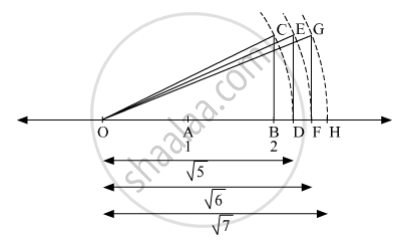# Show the Number √7 on the Number Line. - Mathematics

Sum

Show the number √7 on the number line.

#### SolutionDraw a number line as shown in the figure and mark the points O, A and B on it such that OA = AB = 1 unit. The point O represents 0 and B represents 2. At B, draw CB perpendicular on the number line such that BC = 1 unit. Join OC. Now, ∆OBC is a right angled triangle.
In ∆OBC, by Pythagoras theorem
(OC)2 = (OB)2 + (BC)2
= (2)2 + (1)2
= 4 + 1
= 5
∴ OC = √5
Taking O as centre and radius OC = √5 , draw an arc cutting the number line at D.
Clearly, OC = OD = √5
At D, draw ED perpendicular on the number line such that ED = 1 unit. Join OE. Now, ∆ODE is a right angled triangle.
In ∆ODE, by Pythagoras theorem
(OE)2 = (OD)2 + (DE)2
= (√5)2 + (1)2
= 5 + 1
= 6
∴ OE = √6

Taking O as centre and radius OE = √6 , draw an arc cutting the number line at F.
Clearly, OE = OF = √6
At F, draw GF perpendicular on the number line such that GF = 1 unit. Join OG. Now, ∆OFG is a right angled triangle.
In ∆OFG, by Pythagoras theorem
(OG)2 = (OF)2 + (FG)2
= (√6)2 + (1)2
= 6 + 1
= 7
∴ OG = √7
Taking O as centre and radius OG = √7 , draw an arc cutting the number line at H.
Clearly, OG = OH = √7
Hence, H represents √7 on the number line.

Concept: Rational Numbers on a Number Line
Is there an error in this question or solution?

#### APPEARS IN

Balbharati Mathematics 8th Standard Maharashtra State Board
Chapter 1 Rational and Irrational numbers
Practice Set 1.4 | Q 3 | Page 6